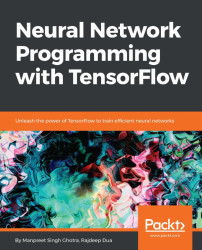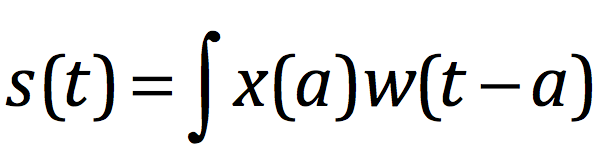•#### Neural Network Programming with Tensorflow#### Overview of this book

If you're aware of the buzz surrounding the terms such as "machine learning," "artificial intelligence," or "deep learning," you might know what neural networks are. Ever wondered how they help in solving complex computational problem efficiently, or how to train efficient neural networks? This book will teach you just that. You will start by getting a quick overview of the popular TensorFlow library and how it is used to train different neural networks. You will get a thorough understanding of the fundamentals and basic math for neural networks and why TensorFlow is a popular choice Then, you will proceed to implement a simple feed forward neural network. Next you will master optimization techniques and algorithms for neural networks using TensorFlow. Further, you will learn to implement some more complex types of neural networks such as convolutional neural networks, recurrent neural networks, and Deep Belief Networks. In the course of the book, you will be working on real-world datasets to get a hands-on understanding of neural network programming. You will also get to train generative models and will learn the applications of autoencoders. By the end of this book, you will have a fair understanding of how you can leverage the power of TensorFlow to train neural networks of varying complexities, without any hassle. While you are learning about various neural network implementations you will learn the underlying mathematics and linear algebra and how they map to the appropriate TensorFlow constructs.
Title PageCreditswww.PacktPub.comCustomer FeedbackPrefaceFree Chapter
Maths for Neural NetworksDeep Feedforward NetworksOptimization for Neural NetworksConvolutional Neural NetworksRecurrent Neural NetworksGenerative ModelsDeep Belief NetworkingAutoencodersResearch in Neural NetworksGetting started with TensorFlow## Convolution operations

Convolution operations are key components of a CNN; these operations use an input tensor and a filter to compute the output. The key is deciding the parameters available to tune them.

Suppose we are tracking the location of an object. Its output is a single x(t), which is the position of the object at time t. Both x and t are real-valued, that is, we can get a different reading at any instant in time. Suppose that our measurement is noisy. To obtain a less noisy estimate of the object's position, we would like to average together measurements. More recent measurements are more relevant for us; we want this to be a weighted average giving higher weight to recent measurements. We can compute this using a weighting function w(a), where a is the age of a measurement (when the measurement was taken) If we apply a weighted average operation at every moment, we obtain a new function providing a smoothed estimate of the position of the object:This operation is called convolution...